Richard Melson

July 2006

Thermodynamics

Thermodynamics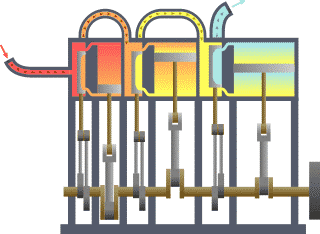Typical thermodynamic system - heat moves from hot (boiler) to cold

(condenser) and work is extracted.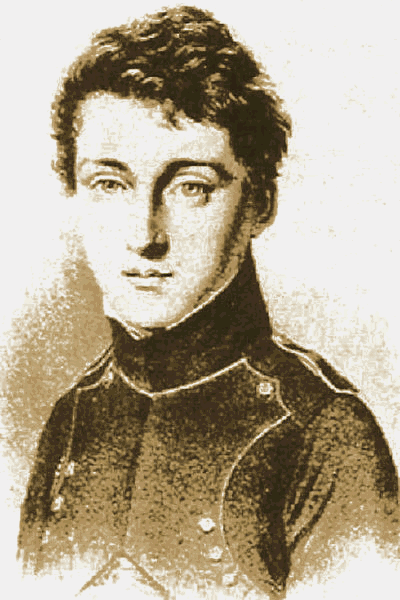Sadi Carnot (1796-1832): the "father" of thermodynamics

## Overview

The starting point for most thermodynamic considerations are the laws of thermodynamics, which postulate that energy can be exchanged between physical systems as heat or work. They also postulate the existence of a quantity named entropy, which can be defined for any system. In thermodynamics, interactions between large ensembles of objects are studied and categorized. Central to this are the concepts of system and surroundings. A system is composed of particles, whose average motions define its properties, which in turn are related to one another through equations of state. Properties can be combined to express internal energy and thermodynamic potentials are useful for determining conditions for equilibrium and spontaneous processes.

With these tools, thermodynamics describes how systems respond to changes in their surroundings. This can be applied to a wide variety of topics in science and engineering, such as engines, phase transitions, chemical reactions, transport phenomena, and even black holes. The results of thermodynamics are essential for other fields of physics and for chemistry, chemical engineering, cell biology, biomedical engineering, and materials science to name a few.

## History

A short history of thermodynamics begins with the German scientist Otto von Guericke who in 1650 built and designed the world's first vacuum pump and created the world's first ever vacuum known as the Magdeburg hemispheres. He was driven to make a vacuum in order to disprove Aristotle's long-held supposition that 'Nature abhors a vacuum'. Shortly thereafter, Irish physicist and chemist Robert Boyle had learned of Guericke's designs and in 1656, in coordination with English scientist Robert Hooke, built an air pump. Using this pump, Boyle and Hooke noticed the pressure-temperature-volume correlation. In time, the ideal gas law was formulated. Then, in 1679, based on these concepts, an associate of Boyle's named Denis Papin built a bone digester, which is a closed vessel with a tightly fitting lid that confines steam until a high pressure is generated.

Later designs implemented a steam release valve to keep the machine from exploding. By watching the valve rhythmically move up and down, Papin conceived of the idea of a piston and cylinder engine. He did not however follow through with his design. Nevertheless, in 1697, based on Papin’s designs, engineer Thomas Savery built the first engine. Although these early engines were crude and inefficient, they attracted the attention of the leading scientists of the time. One such scientist was Sadi Carnot, the "father of thermodynamics", who in 1824 published "Reflections on the Motive Power of Fire", a discourse on heat, power, and engine efficiency. This marks the start of thermodynamics as a modern science.

## Classical thermodynamics

Classical thermodynamics is the original early 1800s variation of thermodynamics concerned with thermodynamic states, and properties as energy, work, and heat, and with the laws of thermodynamics, all lacking an atomic interpretation. In precursory form, classical thermodynamics derives from physicist Robert Boyle’s 1662 postulate that the pressure P of a given quantity of gas varies inversely as its volume V at constant temperature; i.e. in equation form: PV = k, a constant. From here, a semblance of a thermo-science began to develop with the construction of the first successful atmospheric steam engines in England by Thomas Savery in 1697 and Thomas Newcomen in 1712. The first and second laws of thermodynamics emerged simultaneously in the 1850s, primarily out of the works of William Rankine, Rudolf Clausius, and William Thomson (Lord Kelvin). The term thermodynamics was coined by Lord Kelvin in his 1849 publication An Account of Carnot's Theory of the Motive Power of Heat. The first thermodynamic textbook was written in 1859 by William Rankine, a civil and mechanical engineering professor at the University of Glasgow.

## Statistical thermodynamics

With the development of atomic and molecular theories in the late 1800s and early 1900s, thermodynamics was given a molecular interpretation. This field is called statistical thermodynamics, which can be thought of as a bridge between macroscopic and microscopic properties of systems. Essentially, statistical thermodynamics is an approach to thermodynamics situated upon statistical mechanics, which focuses on the derivation of macroscopic results from first principles. It can be opposed to its historical predecessor phenomenological thermodynamics, which gives scientific descriptions of phenomena with avoidance of microscopic details. The statistical approach is to derive all macroscopic properties (temperature, volume, pressure, energy, entropy, etc.) from the properties of moving constituent particles and the interactions between them (including quantum phenomena). It was found to be very successful and thus is commonly used.

## Thermodynamic systems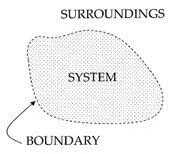An important concept in thermodynamics is the "system". A system is the region of the universe under study. A system is separated from the remainder of the universe by a boundary which may be imaginary or not, but which, by convention delimits a finite volume. The possible exchanges of work, heat, or matter between the system and the surroundings take place across this boundary. There are five dominant classes of systems:

1. Isolated Systems – matter and energy may not cross the boundary.

2. Adiabatic Systems – heat may not cross the boundary.

3. Diathermic Systems - heat may cross boundary.

4. Closed Systems – matter may not cross the boundary.

5. Open Systems – heat, work, and matter may cross the boundary.

For closed systems, as time goes by, internal differences in the system tend to even out; pressures and temperatures tend to equalize, as do density differences. A system in which all equalizing processes have gone practically to completion, is considered to be in a state of thermodynamic equilibrium.

In thermodynamic equilibrium, a system's properties are, by definition, unchanging in time. Systems in equilibrium are much simpler and easier to understand than systems which are not in equilibrium. Often, when analyzing a thermodynamic process, it can be assumed that each intermediate state in the process is at equilibrium. This will also considerably simplify the situation. Thermodynamic processes which develop so slowly as to allow each intermediate step to be an equilibrium state are said to be reversible processes.

## Thermodynamic parameters

The central concept of thermodynamics is that of energy, the ability to do work. As stipulated by the first law, the total energy of the system and its surroundings is conserved. It may be transferred into a body by heating, compression, or addition of matter, and extracted from a body either by cooling, expansion, or extraction of matter. For comparison, in mechanics, energy transfer results from a force which causes displacement, the product of the two being the amount of energy transferred. In a similar way, thermodynamic systems can be thought of as transferring energy as the result of a generalized force causing a generalized displacement, with the product of the two being the amount of energy transferred. These thermodynamic force-displacement pairs are known as conjugate variables. The most common conjugate thermodynamic variables are pressure-volume (mechanical parameters), temperature-entropy (thermal parameters), and chemical potential-particle number (material parameters).

## Thermodynamic instruments

There are two types of thermodynamic instruments, the meter and the reservoir. A thermodynamic meter is any device which measures any parameter of a thermodynamic system. In some cases, the thermodynamic parameter is actually defined in terms of an idealized measuring instrument. For example, the zeroth law states that if two bodies are in thermal equilibrium with a third body, they are also in thermal equilibrium with each other. This principle, as noted by James Maxwell in 1872, asserts that it is possible to measure temperature. An idealized thermometer is a sample of an ideal gas at constant pressure. From the ideal gas law PV=nRT, the volume of such a sample can be used as an indicator of temperature; in this manner it defines temperature. Although pressure is defined mechanically, a pressure-measuring device, called a barometer may also be constructed from a sample of an ideal gas held at a constant temperature. A calorimeter is a device which is used to measure and define the internal energy of a system.

A thermodynamic reservoir is a system which is so large that it does not appreciably alter its state parameters when brought into contact with the test system. It is used to impose a particular value of a state parameter upon the system. For example, a pressure reservoir is a system at a particular pressure, which imposes that pressure upon any test system that it is mechanically connected to. The earth's atmosphere is often used as a pressure reservoir.

It is important that these two types of instruments are distinct. A meter does not perform its task accurately if it behaves like a reservoir of the state variable it is trying to measure. If, for example, a thermometer, were to act as a temperature reservoir it would alter the temperature of the system being measured, and the reading would be incorrect. Ideal meters have no effect on the state variables of the system they are measuring.

## Thermodynamic states

When a system is at equilibrium under a given set of conditions, it is said to be in a definite state. The state of the system can be described by a number of intensive variables and extensive variables. The properties of the system can be described by an equation of state which specifies the relationship between these variables. State may be thought of as the instantaneous quantitative description of a system with a set number of variables held constant.

## Thermodynamic processes

A thermodynamic process may be defined as the energetic evolution of a thermodynamic system proceeding from an initial state to a final state. Typically, each thermodynamic process is distinguished from other processes, in energetic character, according to what parameters, as temperature, pressure, or volume, etc., are held fixed. Furthermore, it is useful to group these processes into pairs, in which each variable held constant is one member of a conjugate pair. The six most common thermodynamic processes are shown below:

1. An isobaric process occurs at constant pressure.

2. An isochoric process, or isometric/isovolumetric process, occurs at constant volume.

3. An isothermal process occurs at a constant temperature.

4. An isentropic process occurs at a constant entropy.

5. An isenthalpic process occurs at a constant enthalpy.

6. An adiabatic process occurs without loss or gain of heat.

## The laws of thermodynamics

In thermodynamics, there are four laws of very general validity, and as such they do not depend on the details of the interactions or the systems being studied. Hence, they can be applied to systems about which one knows nothing other than the balance of energy and matter transfer. Examples of this include Einstein's prediction of spontaneous emission around the turn of the 20th century and current research into the thermodynamics of black holes.

The four laws are:

stating that thermodynamic equilibrium is an equivalence relation.

If two thermodynamic systems are in thermal equilibrium with a third, they are also in thermal equilibrium with each other.

The increase in the energy of a closed system is equal to the amount of energy added to the system by heating, minus the amount lost in the form of work done by the system on its surroundings.

The total entropy of any isolated thermodynamic system tends to increase over time, approaching a maximum value.

As a system asymptotically approaches absolute zero of temperature all processes virtually cease and the entropy of the system asymptotically approaches a minimum value.

See Bose–Einstein condensate and negative temperature.

## Thermodynamic potentials

As can be derived from the energy balance equation on a thermodynamic system there exist energetic quantities called thermodynamic potentials, being the quantitative measure of the stored energy in the system. The four most well known potentials are:

Internal energy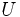Helmholtz free energy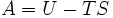Enthalpy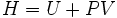Gibbs free energy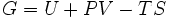Potentials are used to measure energy changes in systems as they evolve from an initial state to a final state. The potential used depends on the constraints of the system, such as constant temperature or pressure. Internal energy is the internal energy of the system, enthalpy is the internal energy of the system plus the energy related to pressure-volume work, and Helmholtz and Gibbs free energy are the energies available in a system to do useful work when the temperature and volume or the pressure and temperature are fixed, respectively.

## References

1. Kelvin, William T. (1849). "An Account of Carnot's Theory of the Motive Power of Heat - with Numerical Results Deduced from Regnault's Experiments on Steam." Transactions of the Edinburg Royal Society, XVI. January 2. Scanned Copy

2. Cengel, Yunus A.; Boles, Michael A. (2005). Thermodynamics - An Engineering Approach. McGraw-Hill. ISBN 0073107689.

1. Perrot, Pierre (1998). A to Z of Thermodynamics. Oxford University Press. ISBN 0198565526.

2. Kroemer, Herbert; Kittel, Charles (1980). Thermal Physics. W. H. Freeman Company. ISBN 0716710889.

3. Goldstein, Martin; Inge, F (1993). The Refrigerator and the Universe. Harvard University Press. ISBN 0674753259.